Next: Degrees of Freedom Up: Deriving the Optimal Scoring Previous: Expected value of the

## Variance of the estimator

The variance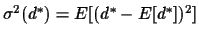can be derived as follows. With the asymptotic value of E[d*] we compute the Taylor series of (d* - E[d*])2 in powers of (w/n - SE(d)). Then we take expected values, replacing the powers of (w/n - SE(d))k by the central moments described in equations 8, 9 and 10. Truncating after two terms we obtain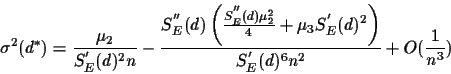The most effective estimator is the one among all possible estimators, which has minimal variance. The variance is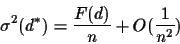where. We can compute E so that it minimizes F(d). This will give us asymptotically (in n) optimal estimators. We have several choices for the minimization: we can derive the best E for
• a given distance d, e.g.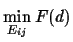.
• a norm for a range of distances, e.g. for d=0 to 200, i.e.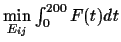.
• the minimax for a range of distances, e.g. for d=0 to 200, i.e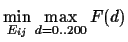.
There is no hope of finding a closed formula for this optimal E, but the first and second cases can be computed numerically without much difficulty.Next: Degrees of Freedom Up: Deriving the Optimal Scoring Previous: Expected value of the
Chantal Korostensky
1999-07-14